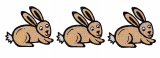# McGraw Hill Math Grade 1 Chapter 1 Lesson 1 Answer Key Counting and Writing from 0 to 5

All the solutions provided in McGraw Hill Math Grade 1 Answer Key PDF Chapter 1 Lesson 1 Counting and Writing from 0 to 5 as per the latest syllabus guidelines.

## McGraw-Hill Math Grade 1 Answer Key Chapter 1 Lesson 1 Counting and Writing from 0 to 5

Counting and Writing from 0 to 5

You can count to find out how many.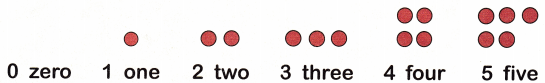Count

Tell how many. Circle the number.

Question 1.There are 3 birds.

Question 2.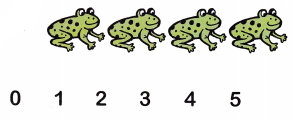From the above figure we observe that there are 4 frogs.

Tell how many. Write the number.

Question 3.Question 4.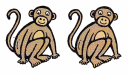_____
There are 2 monkeys in the above figure

Question 5.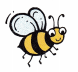________
There is 1 bee in the above figure

Question 6.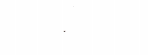________